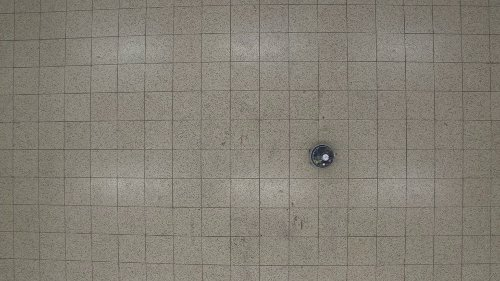# AUTONOMOUS TASK PARTITIONING IN ROBOT FORAGING: AN APPROACH BASED ON COST ESTIMATION

### Supplementary material

by Giovanni Pini, Arne Brutschy, Carlo Pinciroli, Marco Dorigo, and Mauro Birattari
DATE: November 2012

## VIDEO MATERIALThe video shows the trajectory of a foot-bot when both wheel speeds are set to 10 cm/s. In the first part of the video the robots goes backwards, in the second part it goes forwards. The video shows that the trajectory is curved towards the left-hand side with respect to the direction of motion.

## GRIP TIME SAMPLES

The following plot reports the empirical distribution of the time samples used to model object gripping time in simulation. The samples have been collected with real foot-bots performing foraging.

Click on here to open the plot.

This file contains the raw samples. Each value is expressed in control steps (1 step = 0.1 seconds).

TOP

## ODOMETRY ERROR SAMPLES

The following plot reports the odometry error samples that have been collected with real foot-bots and used to build the odometry noise model used in simulation. Each point in the plot reports the X-Y error on a trip from source to nest and back. The closer a point to the origin the smaller the odometry error in the corresponding trip. The dark gray rectangle in the plot representes the nest.

Click on here to open the plot.

TOP

## EXPERIMENTAL SET 1 - Supplementary plots

The goal of the experiments of the firt set is to test the basic properties of the system and to select values for the parameters used in the cost-based selection algorithm. Refer to section 5.1 of the paper for more details about the experiments.

### Effect of noise and swarm size

The plots reported here highlight that the best was of partitioning the transportation task in terms of number of sub-tasks varies with the size of the swarm and with the noise on the odometry system of the robots. The plots report the results of experiments carried out in a 4.5 by 6.7 m arena, with a source-to-nest distance D of 4 meters (refer to Section 4 of the paper). The swarm size is selected in the set {4,6,8,10,15,20}. The odometry noise, expressed as percentage of the default noise, is selected in the set {0%, 20%, 50%, 100%, 120%}.

Effect of noise A first group of plots reports the median of the performance of the fixed algorithm for a given swam size and different values of noise. In general as the noise increases, the peak in performance is obtained in correspondance of a progressively diminishing value of the partition distance characterizing the fixed algorithm. In other words, the higher the noise, the smaller the distance traveled by a robot should be (i.e., the higher the number of sub-tasks the given task should be partitioned into). Click on a link to open the corresponding plot:

4 robots6 robots 8 robots10 robots15 robots20 robots

Effect of the swarm size The following plots report the median of the performance of the fixed algorithm for a given noise factor and different swarm sizes. The plots highlight the effect of interference: for a given noise factor, the peak in performance is obtained, for increasing swarm sizes, by a progressively diminishing value of the partition distance characterizing the fixed algorithm. This indicates that in large swarms task partitioning is advantageous as it allows to physically separate the robots. Click on a link to open the corresponding plot:

No noise20% noise factor50% noise factorstandard noise120% noise factor

Effect of noise and swarmsize, boxplots The following plots report the same data, but plotted as notched boxplot. Each file plots the performance of the fixed partitioning algorithms for a given swarm size and level of noise. Click on a link to open the corresponding plot:

NOISE 0% 4 robots, 6 robots, 8 robots, 10 robots, 15 robots, 20 robots
NOISE 20% 4 robots, 6 robots, 8 robots, 10 robots, 15 robots, 20 robots
NOISE 50% 4 robots, 6 robots, 8 robots, 10 robots, 15 robots, 20 robots
NOISE 100% 4 robots, 6 robots, 8 robots, 10 robots, 15 robots, 20 robots
NOISE 120% 4 robots, 6 robots, 8 robots, 10 robots, 15 robots, 20 robots

TOP

### Performance of the fixed algorithms

The following plot reports the performance of the fixed partitioning algorithms for different swarm sizes and values of the noise factor. The data reported is the same presented in the previous section (see here), reported on the same plot for comparison. Click here to open.

TOP

### Effect of the parameters alpha and epsilon

The following plots report the performance of the cost-base partitioning algorithm obtained with different settings of the parameters alpha and epsilon (refer to Section 3 of the paper for more information). Each plot reports the performance for a given value of epsilon and different values of alpha, number of robots and noise factor. Click on a link to open the corresponding plot:

Epsilon = 0.0Epsilon = 0.05Epsilon = 0.15Epsilon = 0.25Epsilon = 0.5

TOP

### Selected algorithms performance

This plot reports the performance the cost-based partitioning algorithm and of a selection of seven reference algorithms.

TOP

## EXPERIMENTAL SET 3 - Supplementary plots

The goal of the third set of experiments is to test the cost-based partitioning algorithm and the reference algorithms for different values of the source-to-nest distance D. For more information about the third set of experiments refer to section 5.3 of the paper. The following plots report the performance of the algorithms for two values of the source-to-nest distance and for all the tested noise conditions and swarm sizes. Click on a link to open the corresponding plot:

D = 3 meters
D = 6 meters

TOP